# Estimate the Transfer Function of a Circuit with ADALM1000

This example shows how to use MATLAB to connect to an ADALM1000 source-measurement unit, configure it to generate an arbitrary signal, make live measurements, and use the measurements to calculate the transfer function of the connected circuit.

### Introduction

In this example you have an R-C circuit consisting of a 1 k$\Omega$ resistor in series with a 0.1 $\mu$F capacitor. The R-C circuit is attached to the ADALM1000 device with Channel A of the device providing the voltage stimulus consisting of a chirp signal. The output of Channel A is connected to the resistor, and the ground is connected to the capacitor. Channel B of the device is used to measure the voltage across the capacitor. The following circuit diagram shows the measurement setup.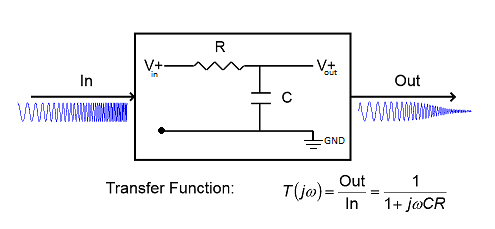You can use the stimulus and the measured waveforms to estimate the transfer function of the R-C circuit, and compare the measured response to the theoretical response of the R-C circuit.

### Discover Devices Connected to Your System

`daqlist("adi")`
```ans=1×4 table DeviceID Description Model DeviceInfo ________ _______________________________ ___________ ________________________ "SMU1" "Analog Devices Inc. ADALM1000" "ADALM1000" [1×1 daq.adi.DeviceInfo] ```

### Create a DataAcquisition for the ADALM1000 Device

`ADIDaq = daq("adi")`
```ADIDaq = DataAcquisition using Analog Devices Inc. hardware: Running: 0 Rate: 100000 NumScansAvailable: 0 NumScansAcquired: 0 NumScansQueued: 0 NumScansOutputByHardware: 0 RateLimit: [] Show channels Show properties and methods ```

### Add Voltage Source and Measurement Channels

The ADALM1000 device is capable of sourcing and measuring voltage simultaneously on separate channels. Set up the device in this mode.

To source voltage, add an analog output channel with device ID SMU1 and channel ID A, and set its measurement type to Voltage.

`addoutput(ADIDaq,'smu1','a','Voltage');`

To measure voltage, add an analog input channel with device ID SMU1 and channel ID B, and set its measurement type to Voltage.

`addinput(ADIDaq,'smu1','b','Voltage');`

Confirm the configuration of the channels.

`ADIDaq.Channels`
```ans=1×2 object Index Type Device Channel Measurement Type Range Name _____ ____ ______ _______ _____________________ _________________ ________ 1 "ao" "SMU1" "A" "Voltage (SingleEnd)" "0 to +5.0 Volts" "SMU1_A" 2 "ai" "SMU1" "B" "Voltage (SingleEnd)" "0 to +5.0 Volts" "SMU1_B" ```

### Define and Visualize a Chirp Waveform for Stimulating the Circuit

Use a chirp waveform of 1 volt amplitude, ranging in frequency from 20 Hz to 20 kHz for stimulating the circuit. The chirp occurs in a period of 1 second.

```Fs = ADIDaq.Rate; T = 0:1/Fs:1; ExcitationSignal = chirp(T,20,1,20e3,'linear');```

Add a DC offset of 2 V to ensure that the output voltage of the device is always above 0 V.

```Offset = 2; ExcitationSignal = ExcitationSignal + Offset;```

Visualize the stimulus signal in the time-domain.

```figure(1) plot(T, ExcitationSignal,'Blue') xlim([0 0.15]) xlabel('Time (s)') ylabel('Magnitude (V)') title('Time domain plot of stimulus signal')```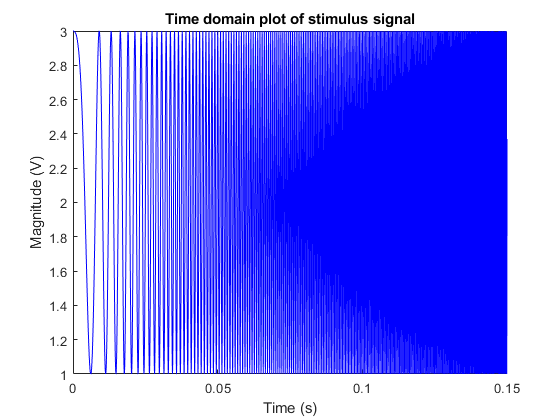Visualize the stimulus signal in the frequency domain.

```figure(2) spectrogram(ExcitationSignal,1024,1000,1024,Fs,'yaxis') title('Frequency domain view of stimulus signal')```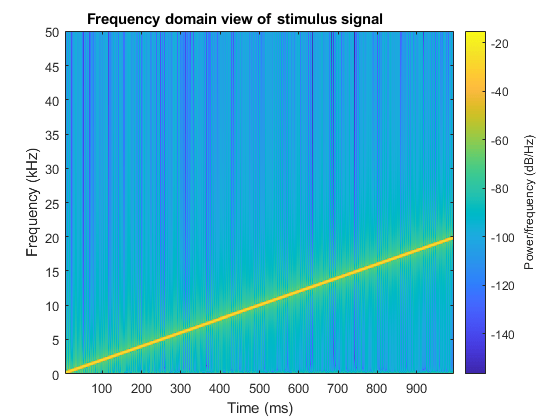### Stimulate the Circuit and Simultaneously Measure the Frequency Response

Generate device output and measure measure the voltage across the capacitor at the same time on the other channel.

```MeasuredTable = readwrite(ADIDaq,ExcitationSignal'); MeasuredSignal = MeasuredTable{:,1};```

### Plot the Stimulus and the Measured Signals

```figure(1) hold on; plot(T,MeasuredSignal,'Red'); xlim([0 0.15]) ylim([1 3]) title('Time domain plot of stimulus and measured signal') legend('Excitation Signal','Measured Signal')```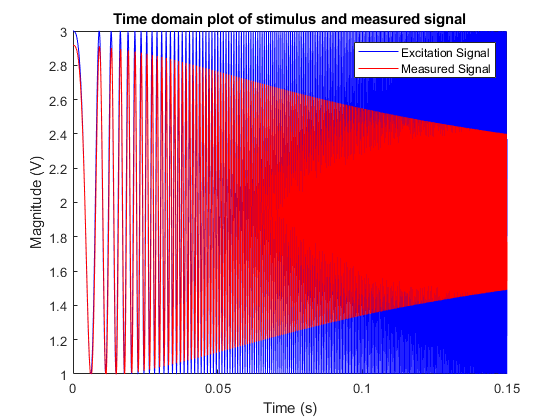```figure(3) spectrogram(MeasuredSignal,1024,1000,1024,Fs,'yaxis') title('Frequency domain view of the the measured signal')```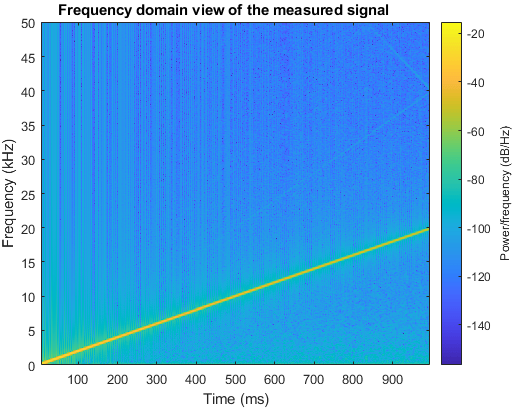### Calculate the Transfer Function of the Circuit

Compare the measured signal and the stimulus signal to calculate the transfer function of the R-C circuit, and plot the magnitude response.

Remove DC offset before processing.

```MeasuredSignal = MeasuredSignal - mean(MeasuredSignal); ExcitationSignal = ExcitationSignal - Offset; [TFxy,Freq] = tfestimate(ExcitationSignal,MeasuredSignal,[],[],[],Fs); Mag = abs(TFxy);```

Compare the estimated transfer function to the theoretical magnitude response.

```R = 1000; % Resistance (ohms) C = 0.1e-6; % Capacitance (farads) TFMagTheory = abs(1./(1 + (1i*2*pi*Freq*C*R))); figure(4); semilogy(Freq,TFMagTheory,Freq,Mag); xlim([0 20e3]) xlabel('Frequency (Hz)') ylim([0.05 1.1]) ylabel('Magnitude') grid on legend('Theoretical frequency response','Measured frequency response') title({'Magnitude response of the theoretical'; 'and estimated transfer functions'});```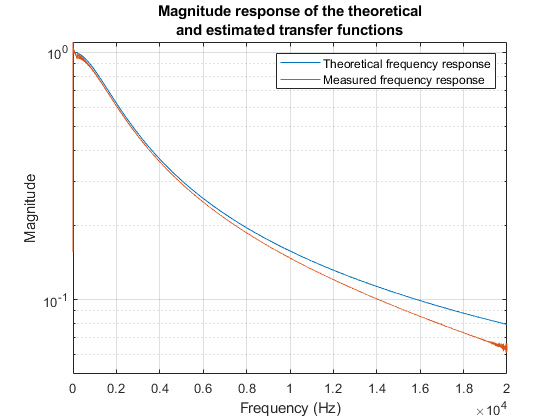### Clear the DataAcquisition and Figures

```clear ADIDaq close all```

## Support